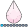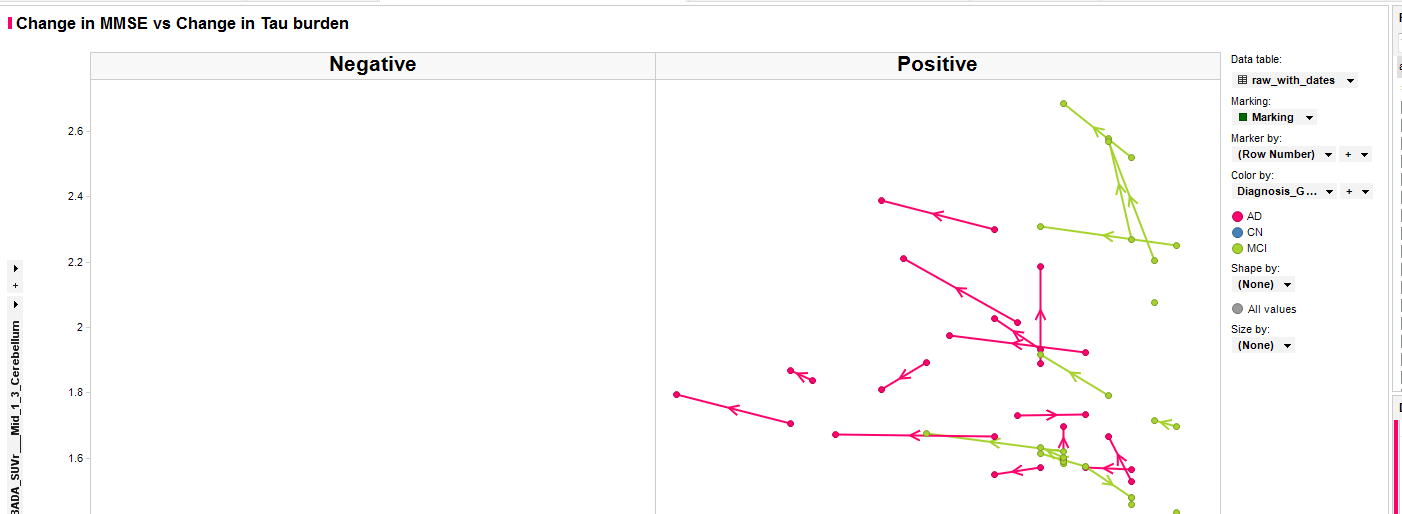djrisks
Barite | Level 11

## Multi-directional arrows on a graph

Hello :-),

I need to replicate the following image below using SAS 9.4, and I was wondering if there were any easier ways to plot the directional arrows? I have thought about using the vector statement because with that I can specify the start and end of the line, and draw the arrow (in the middle) which will be in the right direction, but I was wondering if I could use any other methods please?Kriss

1 ACCEPTED SOLUTION

Accepted Solutions

## Re: Multi-directional arrows on a graph

I think your approach is the best.  If you want to draw the vector from (x1, y1) to (x2, y2), define the midpoint as

m = ( (x1+x2)/2, (y1+y2)/2 ). Then

1. Draw vector from m to (x2, y2). Use the NOARROWHEADS option to suppress the arrow head.

2. In a separate VECTOR statement, draw vector from (x1, y1) to m. Put an arrow head at the end (this is the default).

3. Use the SCATTER statement to overlay the dots at (x1, y1) and (x2, y2).

2 REPLIES 2

## Re: Multi-directional arrows on a graph

I think your approach is the best.  If you want to draw the vector from (x1, y1) to (x2, y2), define the midpoint as

m = ( (x1+x2)/2, (y1+y2)/2 ). Then

1. Draw vector from m to (x2, y2). Use the NOARROWHEADS option to suppress the arrow head.

2. In a separate VECTOR statement, draw vector from (x1, y1) to m. Put an arrow head at the end (this is the default).

3. Use the SCATTER statement to overlay the dots at (x1, y1) and (x2, y2).djrisks
Barite | Level 11

## Re: Multi-directional arrows on a graph

Great! Thank you for this Rick! 🙂

Discussion stats
• 2 replies
• 795 views
• 1 like
• 2 in conversation